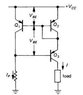# Wilson mirror and Early effect.

Gold Member
Hello all. So I'm currently studying about current mirrors (specifically the Wilson current mirror). I'm reading "The Art of Electronics," page 89 (fig. 2.48 or the image attached). In there it says:
Q1 and Q2 are in the usual mirror configuration, but Q3 now keeps Q1's collector fixed at two diode drops below Vcc. That circumvents the Early effect in Q1, whose collector is now the programming terminal, with Q2 now sourcing the output current.

Ok so most of this makes sense to me, but shouldn't Q3 be the one "circumvented" from the Early effect since the load is attached to it?

#### Attachments

•wilson mirror.jpg
5.7 KB · Views: 1,171

Hello all. So I'm currently studying about current mirrors (specifically the Wilson current mirror). I'm reading "The Art of Electronics," page 89 (fig. 2.48 or the image attached). In there it says:

Ok so most of this makes sense to me, but shouldn't Q3 be the one "circumvented" from the Early effect since the load is attached to it?

The thing is, the whole idea of a current source/sink is that it should be able to work into a wide range of voltages while maintaining a constant current. So we can't avoid having collector voltage variations at Q3, to do so would "circumvent" the very purpose of a current source.

What's different about Q3 in the Wilson current mirror compared to the basic two transistor current source is that it is the emitter current that is being "mirrored" in the Wilson case (@ Q3) whereas it is effectively the base current that's being mirrored in the simpler two transistor case. You'll notice that the input to Q3 (output of the Q1 Q2 mirror) is on the emitter and the output on the collector. So Q3 is effectively a common base stage buffering the Q1/Q2 mirror.

This make a huge difference to it's susceptibility to Early effect. See my reply #16 in the following post for further explanation of why this is so. https://www.physicsforums.com/showthread.php?t=634511

Last edited:
Gold Member

What I understand from what you are saying is that "circumventing" the Early effect for Q3 defeats the purpose of a current source. Why? Because that will cancel the negative feedback from the load. Is that correct? I have also learned from your post #16 that the Early effect is not noticeable in a common base configuration (I need to think about this some more).

I don't understand how in a regular current mirror it is the base current that is being mirrored as you have stated. I thought the input current is being mirrored to the load?

I don't understand how in a regular current mirror it is the base current that is being mirrored as you have stated. I thought the input current is being mirrored to the load?

In a normal two transistor current mirror, they are connected so that the base emitter junction voltages are identical for each transistor (and the current in one of them is well defined by the external resistance/voltage).

Since the transistors are matched, and are supplied with identical base-emitter voltages, the theory is that they must therefore have identical base currents. Now if the current gains ($\beta$) are matched then it follows that collector currents will also be identical.

The problem is however, that the Early effect causes beta (of the output transistor) to increase as Vce increases, due to base narrowing. In the Wilson mirror this is countered by allowing the base matched pair Q1/Q2 to operate at similar Vce levels and buffering the output through Q3 (so as to allow for voltage swings at the Q3 collector).

Now Q3 will also be subject to the Early effect (base narrowing and increased current gain), but since Q3 is common base we are only interested in the ratio of Ic/Ie instead of the ratio Ic/Ib as per Q1/Q2.

And since $I_c/I_e = \frac{\beta}{\beta + 1}$, it is largely unaffected by small or even moderate changes in beta. (See numerical example in previously linked post).

Last edited:
Gold Member
Thank you for your reply and help. You have not answered one of my response questions. Is this correct?
What I understand from what you are saying is that "circumventing" the Early effect for Q3 defeats the purpose of a current source. Why? Because that will cancel the negative feedback from the load.